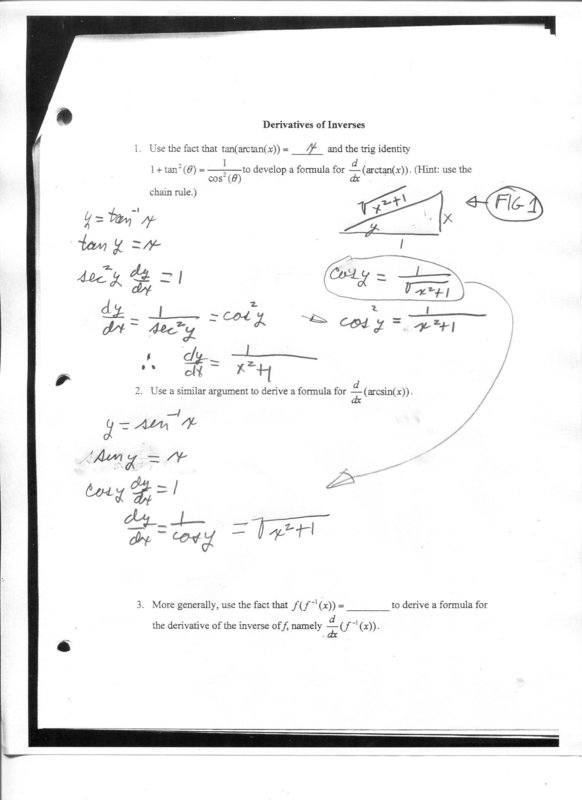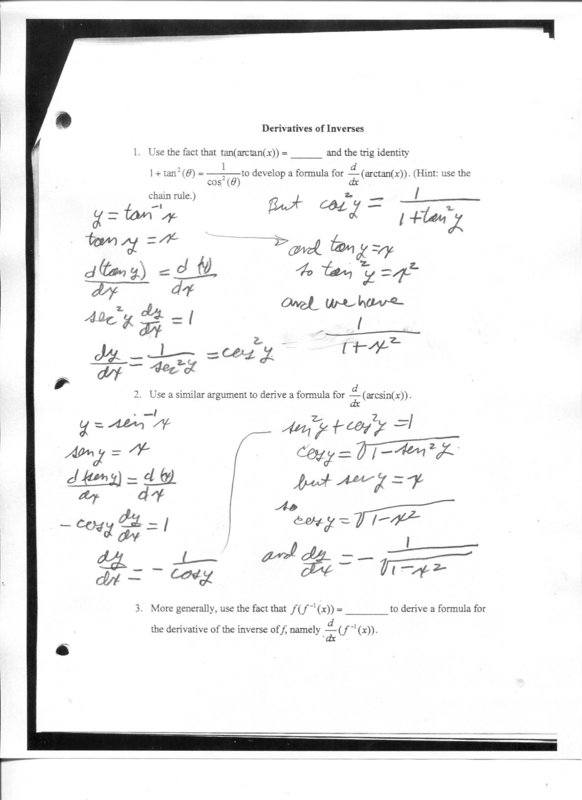# How do I calculate the derivative of the inverse sin and inverse tan

Homework Statement:
I need to calculate the expression for the derivative of the inverse tan and sin. I have written my solution of the inverse tan on the attachment but I have questions. Also, using the same method, the calculation of the derivative of the inverse sin does not work.
Relevant Equations:
y = tan^-1(x) find dy/dx and y = sin^-1(x) find dy/dx
I calculated an expression for the derivative of the inverse tan but I did not use the identity as suggested. Why did I need to use this identity. Did I do the problem correctly? I got the correct answer.

I tried to do the derivative of the inverse sin the same way. I used the same figure 1 on the diagram that I used for the tan problem. However, my answer, sqrt(x^2 + 1) is not correct, The correct answer, according to the book is 1/sqrt(1-x^2).

I cannot see the error.## Answers and Replies

WWGD
Science Advisor
Gold Member
If f and g are inverses of each other ,do you know what f(g(x)) is? Do you know how to use the chain rule here?

Yes, f(g(x)) = x if they are inverses.

I have found an explanation of these inverses that do not use the triangles I used in the original post. I thought the triangles would help but it seems not to be necessary. Even though I have the solution, Iwould still like to know why the inverse sin I calculated in the original post is incorrect.hutchphd
Science Advisor
Homework Helper
Using the picture method for sin one would draw a triangle with hypotenuse =1

I agree hutch but is there some reason I could not use the fig 1 diagram?

hutchphd
Science Advisor
Homework Helper
The sin of y is not x. It is x/ hypotenuse. You need to use it correctly!

Agreed, but I would think that since both diagrams are correct in a Pythagorean sense that using a change of variable or other trick might make the first figure work. I guess not. I have the correct answer now I will just have ot clear my head. Thanks

hutchphd
Science Advisor
Homework Helper
If you do it correctly it will work perfectly. If you write down an equation that is incorrect you will get wrong anwers. From your picture, write down sin (y). Proceed. It will be messy.

WWGD
Science Advisor
Gold Member
For one,d/dx(sinx)=cosx, not -cosx. And when setting y=tan−1(x)y=tan−1(x) you do not get d(y)/dx=1. Nice try, but as Hutch said, you need to mind the details.

WWDG, you are correct. With the fixed error, the answer would be correct.

•berkeman, hutchphd and WWGD